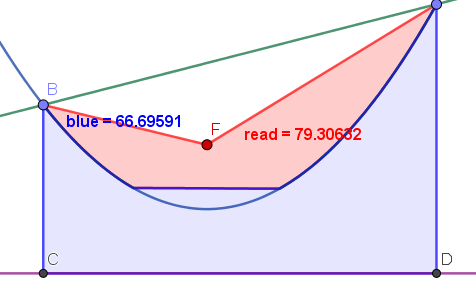# Parabolic area

Dmitry Ezhov shared this question 2 years ago

Parabola p created by tool "Parabola" by focus F and directrix d. Points A and B lying on p and set line h. How get area betwen p and h? Command "IntegralBetween(p,h,x(A),x(B))" not work with error "Illegal argument: Parabola p".

Files: test.ggb1

The Integral command works with functions.

And your parabola is recognized by GeoGebra as a conic, moreover in implicit form.

You need to use functional notation like e.g. F(x)=x^2+2x+1 in order to us the related commands11

An aproximative surface is possible (in any position)

It helps to draw the area graphically and give a aproximative value to check the funcionaly formula.1

Ok. How make b (polygon ABF) with parabolic segment a in one color, and c (polygon ABCD) without a in other color?1

it's alway the same principle.1

Bug in Segment?1

Yes, this solution has a restriction.1

`RightSide(p) <= LeftSide(p) && (x-x(A))*(y(F)-y(A))-(y-y(A))*(x(F)-x(A)) <= 0 && (x-x(F))*(y(B)-y(F))-(y-y(F))*(x(B)-x(F)) <= 0`

and

`RightSide(p) >= LeftSide(p) && (x-x(A))*(y(D)-y(A))-(y-y(A))*(x(D)-x(A)) >= 0 && (x-x(C))*(y(B)-y(C))-(y-y(C))*(x(B)-x(C)) >= 0 && y>= y(C)`
or

`Polygon(Join({{F}, Sequence(Point(p, k), k, PathParameter(A), PathParameter(B), (PathParameter(B) - PathParameter(A)) / 50)}))`
and

`Polygon(Join({{C, D}, Sequence(Point(p, k), k, PathParameter(A), PathParameter(B), (PathParameter(B) - PathParameter(A)) / 50)}))`1

the AREA with great accuracy

Files: foro.ggb1

theory in https://en.wikipedia.org/wi...

and the graph attached

Files: foro.ggb1

Thanks!

If parabola set parametric, how get separate 0.53t^2-4.55t-2.92 and 2.28t^2+1.06t-0.35?1

Activity without integration.

How set restriction corner_AFB<180 degrees?1

see the dynamic definition of H

Files: foro.ggb